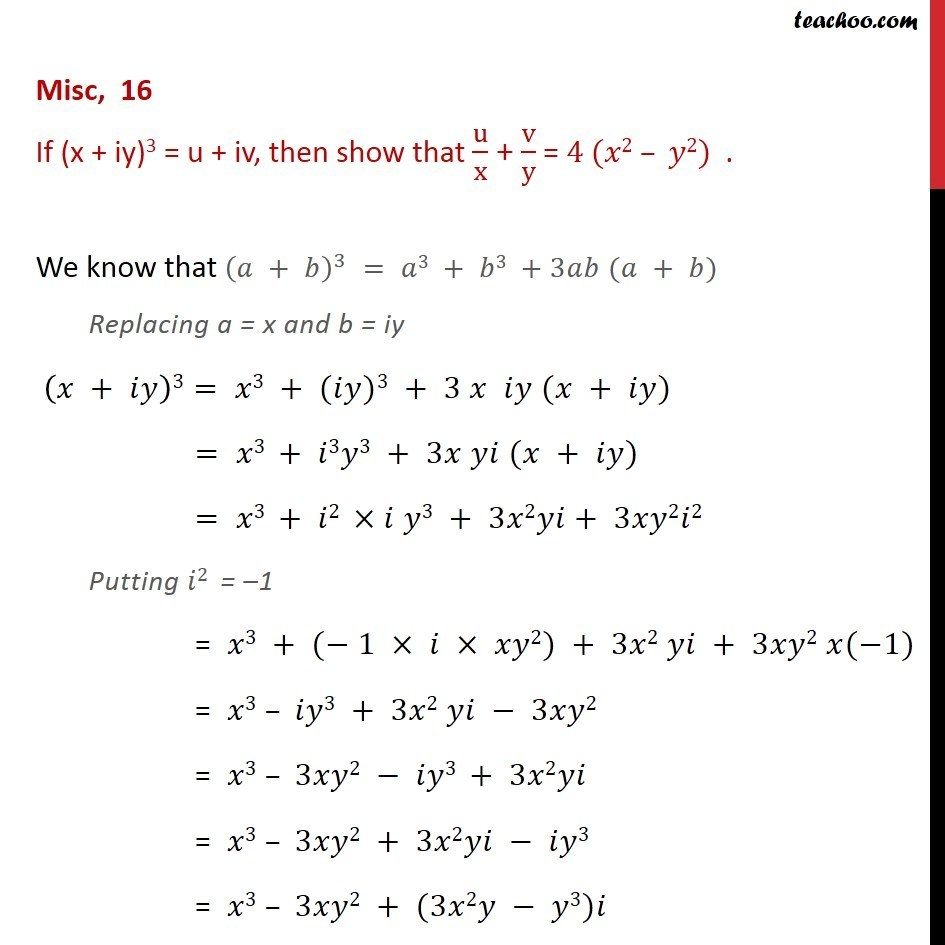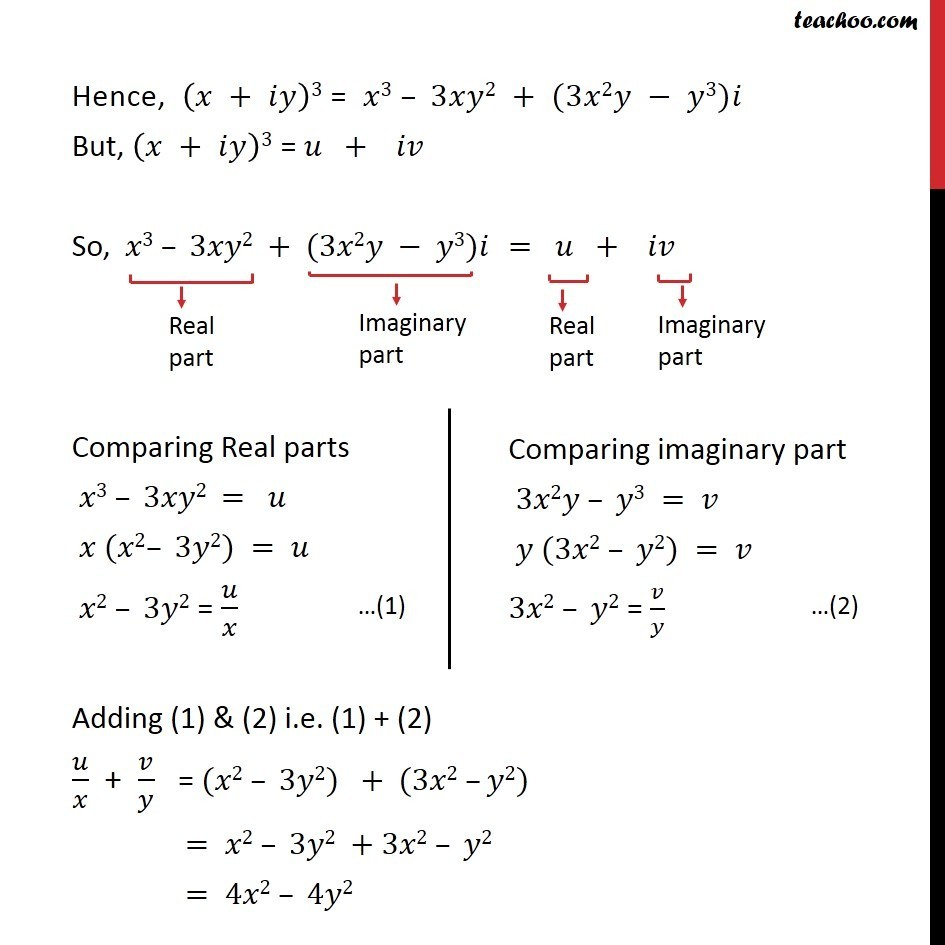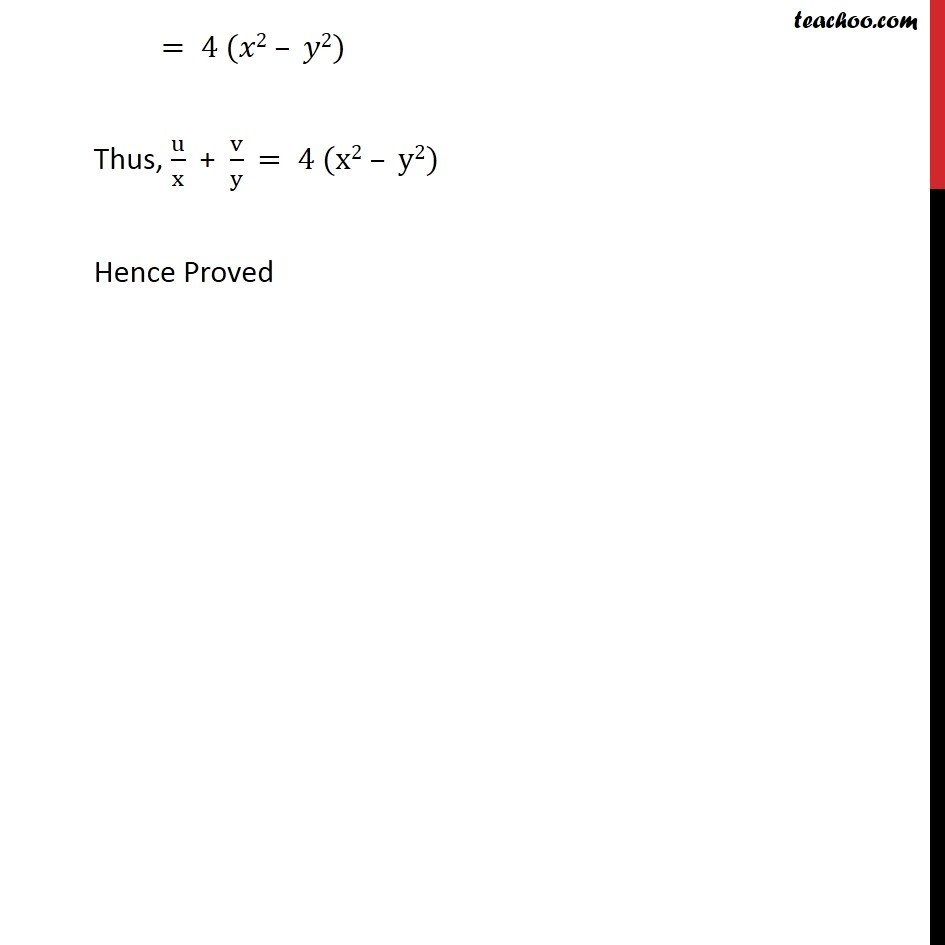Proof- Solving

Chapter 5 Class 11 Complex Numbers (Term 1)
Concept wise### Transcript

Misc, 16 If (x + iy)3 = u + iv, then show that u/x + v/y = 4 (𝑥2 – 𝑦2) . We know that (𝑎 + 𝑏)^3 = 𝑎3 + 𝑏3 +3𝑎𝑏 (𝑎 + 𝑏) Replacing a = x and b = iy (𝑥 + 𝑖𝑦)3= 𝑥3 + (𝑖𝑦)3 + 3 𝑥 𝑖𝑦 (𝑥 + 𝑖𝑦) = 𝑥3 + 𝑖3𝑦3 + 3𝑥 𝑦𝑖 (𝑥 + 𝑖𝑦) = 𝑥3 + 𝑖2 ×𝑖 𝑦3 + 3𝑥2𝑦𝑖+ 3𝑥𝑦2𝑖2 Putting 𝑖2 = –1 = 𝑥3 + (− 1 × 𝑖 × 𝑥𝑦2) + 3𝑥2 𝑦𝑖 + 3𝑥𝑦2 𝑥(−1) = 𝑥3 – 𝑖𝑦3 + 3𝑥2 𝑦𝑖 − 3𝑥𝑦2 = 𝑥3 – 3𝑥𝑦2 − 𝑖𝑦3 + 3𝑥2𝑦𝑖 = 𝑥3 – 3𝑥𝑦2 + 3𝑥2𝑦𝑖 − 𝑖𝑦3 = 𝑥3 – 3𝑥𝑦2 + (3𝑥2𝑦 − 𝑦3)𝑖 Hence, (𝑥 + 𝑖𝑦)3 = 𝑥3 – 3𝑥𝑦2 + (3𝑥2𝑦 − 𝑦3)𝑖 But, (𝑥 + 𝑖𝑦)3 = 𝑢 + 𝑖𝑣 So, 𝑥3 – 3𝑥𝑦2 + (3𝑥2𝑦 − 𝑦3)𝑖 = 𝑢 + 𝑖𝑣 Comparing Real parts 𝑥3 – 3𝑥𝑦2 = 𝑢 𝑥 (𝑥2– 3𝑦2) = 𝑢 𝑥2 – 3𝑦2 = 𝑢/𝑥 Adding (1) & (2) i.e. (1) + (2) 𝑢/𝑥 + 𝑣/𝑦 = (𝑥2 – 3𝑦2) + (3𝑥2 –𝑦2) = 𝑥2 – 3𝑦2 +3𝑥2 – 𝑦2 = 4𝑥2 – 4𝑦2 = 4 (𝑥2 – 𝑦2) Thus, u/x + v/y = 4 (x2 – y2) Hence Proved